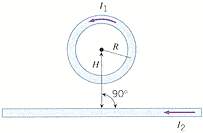# Magnetic Fields Produced by Currents

• Dart82
In summary, the problem involves finding the distance H in terms of the radius R of a circular loop of wire and a long, straight wire carrying currents of I1 and I2 respectively. Using the equations for the magnetic field produced by a long straight wire and at the center of a loop, it can be determined that H is equal to the radius R.

## Homework Statement

A circular loop of wire and a long, straight wire carry currents of I1 and I2 (see the drawing), where I2 = 5.2I1. The loop and the straight wire lie in the same plane. The net magnetic field at the center of the loop is zero. Find the distance H, expressing your answer in terms of R, the radius of the loop.## Homework Equations

Magnetic field produced by a long straight wire:
B = (u*I)/2 pi r

Magnetic field at center of a loop:
B = N * [(u*I)/(2R)]

## The Attempt at a Solution

honestly i don't know where to begin. I am not getting how the equations i listed will help me to solve for H in terms of R. obviously, i know i have to solve for R in order to find H. I'm confused as to which equation to use. id also assume some type of triangle will come into play here since i am given a right angle. If someone could please help me get going in the right direction on this one i would greatly appreciate it..

Magnetic field produced by a long straight wire:
B = (u*I)/2 pi r
The r in this equation is equal to H. You also know that the wire's field has to cancel out the loop's field. Proceed from there.

i was just about to post this big long reply...but i finally got it! thanks